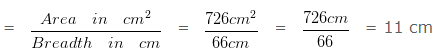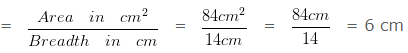# To Find Perimeter of Rectangle when Area & Breadth given

Question 1

The area of a rectangle is 726 cm² and its breadth is 66 cm. Find the perimeter of the rectangle?

Explanation

Given

Area = 726 cm²

Length of the rectanglePerimeter of the rectangle = 2 x ( l + b ) cm

= 2 x ( 66 + 11 ) cm

= ( 2 x 77 ) cm

= 154 cm

Hence, the perimeter of the rectangle is 154 cm

Question 2

The area of a rectangle is 84 cm² and its breadth is 14 cm. Find the perimeter of the rectangle?

Explanation

Given

Area = 84 cm²

Length of the rectanglePerimeter of the rectangle = 2 x ( l + b ) cm

= 2 x ( 14 + 6 ) cm

= ( 2 x 20 ) cm

= 40 cm

Hence, the perimeter of the rectangle is 40 cm